# One Page Summary: “PaxosStore: High-availability Storage Made Practical in WeChat”

PaxosStore paper, published in VLDB 2017, describes the large scale, multi-datacenter storage system used in WeChat. As the name may suggest, it uses Paxos to provide storage consistency. The system claims to provide storage for many components of the WeChat application, with 1.5TB of traffic per day and tens of thousands of queries per second during the peak hours.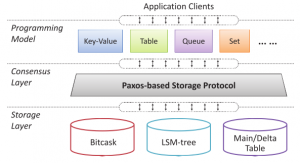PaxosStore relies on Paxos protocol to for consistency and replication within tight geographical regions. The system was designed with a great separation of concerns in mind. At a high level, it has three distinct layers interacting with each other: API layer, consensus layer, and storage.  Separating these out allowed PaxosStore provide most suitable APIs and storage for different tasks and application, while still having the same Paxos-backed consistency and replication.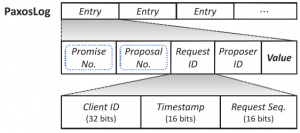In a paxos-driven consensus layer,  the system uses a per-object log to keep track of values and paxos-related metadata, such as promise (epoch) and proposal (slot) numbers. Log’s implementation, however, seems to be somewhat decoupled from the core Paxos protocol. Paxos implementation is leaderless, meaning there are no single dedicated leader for each object, and every node can perform writes on any of the objects in the cluster by running prepare and accept phases of Paxos. Naturally, the system tries to perform (most) writes in one round trip instead of two by assuming some write locality. After the first successful write, a node can issue more writes with increasing proposal (slot) numbers. If some other node performs a write, it needs to have higher ballot, preventing the old master from doing quick writes. This is a rather common approach, used in many Paxos variants.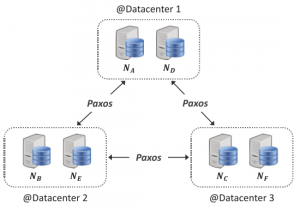PaxosStore runs in multiple datacenters, but it is not a full-fledged geo-replicated system, as it only replicates between the datacenters located in the same geographical area. The paper is not clear on how data get assigned to regions and whether objects can migrate between regions in any way. Within each datacenter the system organizes nodes into mini-clusters, with each mini-cluster acting as a Paxos follower. When data is replicated between mini-clusters, only one (some?) nodes in each mini-cluster hold the data. This design aims to improve fault tolerance: with a 2-node mini-cluster, failure of 1 node does not result in the failure of the entire mini-cluster Paxos-follower.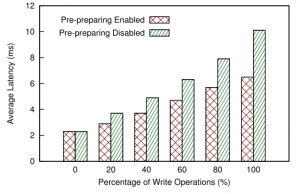The paper somewhat lacks in its evaluation, but PaxosStore seems to handle its goal of multi-datacenter, same-region replication fairly well, achieving sub-10 ms writes.

This paper seems like a good solution for reliable and somewhat localized data-store. The authors do not address data sharding and migration between regions and focus only on the intra-region replication to multiple datacenters, which makes me thing PaxosStore is not really “global”, geo-replicated database.  The fault tolerance is backed by Paxos, mini-clusters and the usage of PaxosLog for data recovery. The evaluation could have been more complete if authors showed scalability limits of their system and provided some details about throughput and datacenter-locality of the workload in the latency experiments.

Here is one page pdf of this summary.

# Modeling Paxos Performance in Wide Area – Part 3

Earlier I looked at modeling paxos performance in local networks, however nowadays people (companies) use paxos and its flavors in the wide area as well. Take Google Spanner and CockroachDB as an example. I was naturally curious to expand my performance model into wide area networks as well. Since our lab worked on WAN coordination for quite some time, I knew what to expect from it, but nevertheless I got a few small surprises along the way.

In this post I will look at Paxos over WAN, EPaxos and our wPaxos protocols. I am going to skip most of the explanation of how I arrived to the models, since the models I used are very similar in spirit to the one I created for looking at local area performance. They all rely on queuing theory approximations for processing overheads and k-order statistics for impact of quorum size.

Despite being similar in methods used, modeling protocols designed for WAN operation proved to be more difficult than local area models. This difficulty arises mainly from the myriad of additional parameters I need to account for. For instance, for Paxos in WAN I need to look at latencies between each node in the cluster, since the WAN-networks are not really uniform in inter-region latencies. Going up to EPaxos, I have multiple leaders to model, which means I also must take into consideration the processing overheads each node takes in its role of following other nodes for some slots. wPaxos takes this even further: to model its performance I need to consider access locality and “object stealing” among other things.

Today I will focus only on 5 region models. In particular, I obtained average latencies between 5 AWS regions: Japan (JP), California (CA), Oregon (OR), Virginia (VA) and Ireland (IR). I show these regions and the latencies between them in Figure 1 below.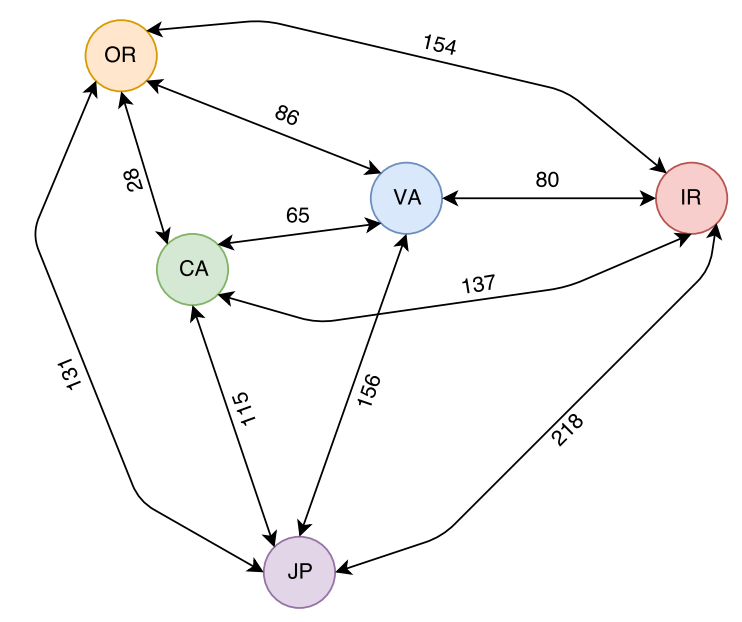Figure 1: Some AWS regions and latencies in ms between them.

## Paxos in WAN

Converting paxos model from LAN to WAN is rather straightforward; all I need to do is to modify my paxos model to take non-uniform distances between nodes. I also need the ability to set which node is going to be the leader for my multi-paxos rounds. With these small changes, I can play around with paxos and see how WAN affects it.

Figure 2 (above) shows a model run for 5 nodes in 5 regions (1 node per region). From my previous post, I knew that the maximum throughput of the system does not depend on network latency, so it is reasonable for paxos in WAN to be similar to paxos in local networks in this regard. However, I was a bit surprised to see how flat the latency stays in WAN deployment almost all the way till reaching the saturation point. This makes perfect sense, however, since the WAN RTT dominates the latency and small latency increases due to the queuing costs are largely masked by large network latency. This also may explain why Spanner, CockroachDB and others use paxos in databases; having predictable performance throughout the entire range of load conditions makes it desirable for delivering stable performance to clients and easier for load-balancing efforts.

However, not everything is so peachy here. Geographical placement of the leader node plays a crucial role in determining the latency of the paxos cluster. If the leader node is too far from the majority quorum nodes, it will incur high latency penalty. We see this with Japan and Ireland regions, as they appear far from all other nodes in the system and result in very high operation latency.

## EPaxos

EPaxos protocol tries to address a few shortcoming in paxos. In particular, EPaxos no longer has a single leader node and any node can lead some commands. If commands are independent, then EPaxos can commit them quickly in one phase using a fast quorum. However, if the command have dependencies, EPaxos needs to run another phase on a majority quorum (at which point it pretty much becomes Paxos with two phases for leader election and operation commit). The fast quorum in some cases may be larger than the majority quorum, but in the 5-node model I describe today, the fast quorum is the same as the majority quorum (3 nodes).

Naturally, conflict between commands will impact the performance greatly: with no conflict, all operations can be decided in one phase, while with 100% conflict, all operations need two phases. Since running two phases requires more messages, I had to change the model to factor in the probability of running two phases. Additionally, the model now looks at the performance of every node separately, and account for the node leading some slots and following on the other.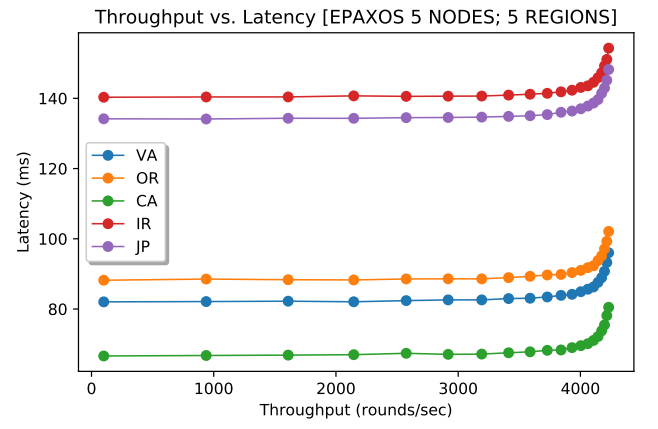Figure 3: EPaxos throughput at every node with 2% command conflict.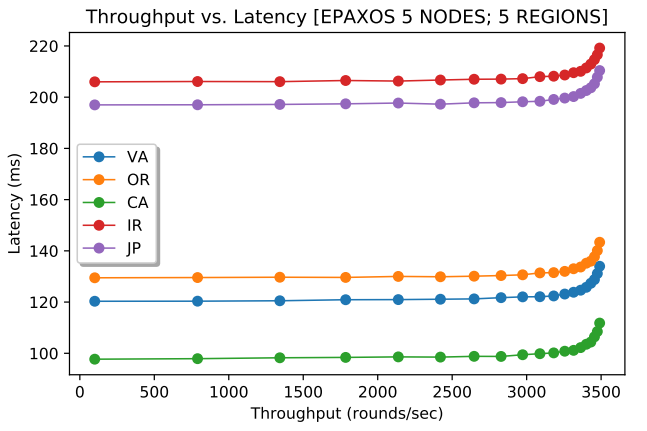Figure 4: EPaxos throughput at every node with 50% command conflict.

Figures 3 and 4 (above) show EPaxos performance at every node for 2% and 50% conflict. Note that the aggregate throughput of the cluster is a sum of all 5 nodes. For 2% conflict, the max throughput was 2.7 times larger than that of Paxos. As the conflict between commands increases, EPaxos loses its capacity and its maximum throughput decreases, as I illustrate in Figure 5 (below). This changing capacity may make more difficult to use EPaxos in production environments. After all, workload characteristics may fluctuate throughout the system’s lifespan and EPaxos cluster may or may not withstand the workloads of identical intensity (same number of requests/sec), but different conflict.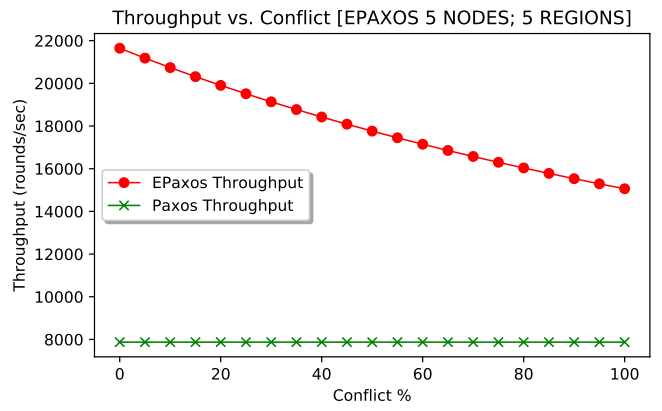Figure 5: EPaxos aggregate maximum throughput for different conflict levels.

## wPaxos

wPaxos is our recent flavor of WAN-optimized paxos. Its main premise is to separate the commands for different entities (objects) to different leaders and process these commands geographically close to where the entities are required by clients. Unlike most Paxos flavors, wPaxos needs large cluster, however, thanks to flexoble quorums, each operations only uses a subset of nodes in the cluster. This allows us to achieve both multi-leader capability and low average latency.

wPaxos, however, has lots of configurable parameters that all affect the performance. For instance, the fault tolerance may be reduced to the point where a system does not tolerate a region failure, but can still tolerate failure of nodes within the region. In this scenario (Figure 6, below), wPaxos can achieve the best performance with aggregate throughput across all regions (and 3 nodes per region for a total of 15 nodes) of 153,000 requests per second.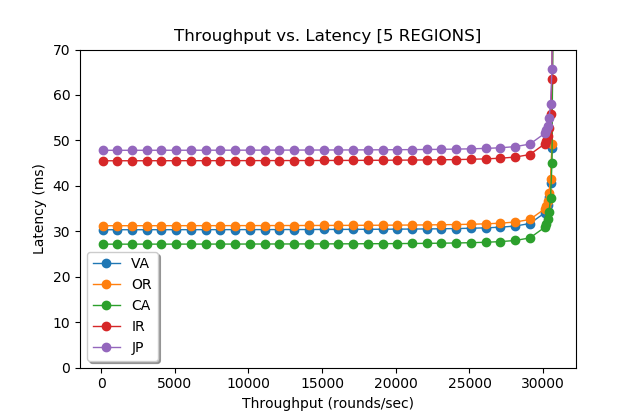Figure 6: wPaxos with no region fault tolerance and 70% region-local operations and 1% chance of object migration.

We still observe big differences in latencies due to the geography, as some requests originating in one regions must go through stealing phase or be resolved in another region. However, the average latency for a request is smaller than that of EPaxos or Paxos. Of course, a direct comparison between wPaxos and EPaxos is difficult, as wPaxos (at least in this model configuration) is not as fault tolerant as EPaxos. Also unlike my FPaxos model from last time, wPaxos model also reduces the communication in phase-2 to a phase-2 quorum only. This allows it to take much bigger advantage of flexible quorums than “talk-to-all-nodes” approach. As a result, having more nodes helps wPaxos provide higher throughput than EPaxos.
Some EPaxos problems still show-up in wPaxos. For instance, as the access locality decreases and rate of object migration grows, the maximum throughput a cluster can provide decreases. For instance, Figure 7 (below) shows wPaxos model with locality shrunken to 50% and object migration expanded to 3% of all requests.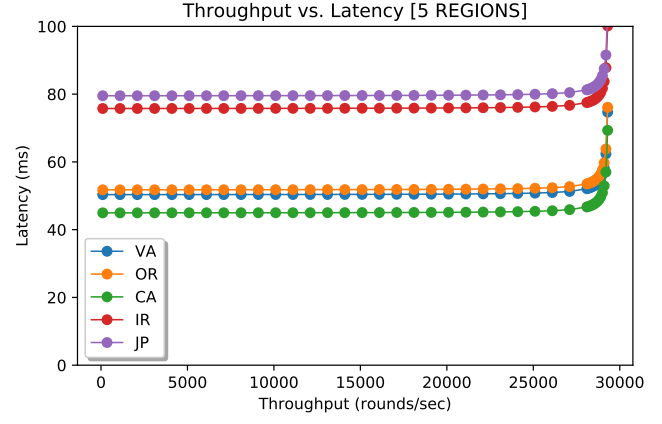Figure 7: wPaxos with no region fault tolerance and 50% region-local operations and 3% chance of object migration.

## How Good Are the Models?

I was striving to achieve the best model accuracy without going overboard with trying to account all possible variables in the model. The models both for LAN and WAN seems to agree fairly well with the results we observe in our Paxi framework for studying various flavors of consensus.

However, there is always room for improvement, as more parameters can be accounted for to make more accurate models. For instance, WAN RTTs do not really follow a single normal distribution, as a packet can take one of many routes from one region to another (Figure 8, below). This may make real performance fluctuate and “jitter” more compared to a rather idealistic model.

I did not account for some processing overheads as well. In EPaxos, a node must figure out the dependency graph for each request, and for high-conflict workloads these graphs may get large requiring more processing power. My model is simple and assumes this overhead to be negligible.

## Few Concluding Remarks

Over the series of paxos performance modeling posts I looked at various algorithms and parameters that affect their performance. I think it truly helped me understand Paxos a bit better than before doing this work. I showed that network fluctuations have little impact on paxos performance (k-order statistics helps figure this one out). I showed how node’s processing capacity limits the performance (I know this is trivial and obvious), but what is obvious, but still a bit interesting about this is that a paxos node processes roughly half of the messages that do not make a difference anymore. Once the majority quorum is reached, all other messages for a round carry a dead processing weight on the system.

The stability of Paxos compared to other more complicated flavors of paxos (EPaxos, wPaxos) also seems interesting and probably explains why production-grade systems use paxos a lot. Despite having lesser capacity, paxos is very stable, as its latency changes little at levels of throughput. Additionally, The maximum throughput of paxos is not affected by the workload characteristics, such as conflict or locality. This predictability is important for production systems that must plan and allocate resources. It is simply easier to plan for a system delivering stable performance regardless of the workload characteristics.

Geography plays a big role in WAN paxos performance. Despite the cluster having the same maximum throughput, the clients will observe the performance very differently depending on the leader region. Same goes with EPaxos and wPaxos, as different regions have different costs associated with communicating to the quorums, meaning that clients in one region may observe very different latency than their peers in some other regions. I think this may make it more difficult to provide same strong guarantees (SLAs?) regarding the latency of operations to all clients in production systems.

There are still many things one can study with the models, but I will let it be for now. Anyone who is interested in playing around may get the models on GitHub.

# Modeling Paxos Performance – Part 2

In the previous posts I started to explore node-scalability of paxos-style protocols. In this post I will look at processing overheads that I estimate with the help of a queue or a processing pipeline. I show how these overheads cap the performance and affect the latency at different cluster loads.

I look at the scalability for a few reasons. For one, in the age of a cloud 3 or 5 nodes cluster may not be enough to provide good resilience, especially in environments with limited control over the node placement. After all, a good cluster needs to avoid nodes that share common points of failures, such as switches of power supply. Second, I think it helps me learn more about paxos and its flavors and why certain applications chose to use it.  And third, I want to look at more exotic paxos variants and how their performance may be impacted by different factors, such as WAN or flexible quorums. For instance, flexible quorums present the opportunity to make trade-offs between performance and resilience. We do this by adjusting the sizes of quorums for phase-1 and phase-2. This is where the modeling becomes handy, as we can check if a particular quorum or deployment makes a difference from the performance standpoint.

Last time, I looked at how local network variations affect the performance when scaling the cluster up in the number of servers. What I realized is that the fluctuations in message round-trip-time (RTT) can only explain roughly 3% performance degradation going from 3 nodes to 5, compared to 30-35% degradation in our implementation of paxos. We also see that this degradation depends on the quorum size, and for some majority quorum deployments there may even be no difference due to the network. In this post I improve the model further to account for processing bottlenecks.

As a refresher from the previous time, I list some of the parameters and variables I have been using:

• $$l$$ – some local message in a round
• $$r_l$$ – message RTT in local area network
• $$\mu_l$$ – average message RTT in local area network
• $$\sigma_l$$ – standard deviation of message RTT in local area network
• $$N$$ – number of nodes participating in a paxos phase
• $$q$$ – Quorum size. For a majority quorum $$q=\left \lfloor{\frac{N}{2}}\right \rfloor +1$$
• $$m_s$$ – time to serialize a message
• $$m_d$$ – time to deserialize and process a message
• $$\mu_{ms}$$ – average serialization time for a single message
• $$\mu_{md}$$ – average message deserialization time
• $$\sigma_{ms}$$ – standard deviation of message serialization time
• $$\sigma_{md}$$ – standard deviation of message deserialization time

The round latency $$L_r$$ of was estimated by $$L_r = m_s + r_{lq-1} + m_d$$, where $$r_{lq-1}$$ is the RTT + replica processing time for the $$q-1$$th fastest messages $$l_{q-1}$$

## Message Processing Queue

Most performance difference in the above model comes from the network performance fluctuations, given that $$m_s$$, $$m_d$$ and their variances are small compared to network latency. However, handling each message creates significant overheads at the nodes that I did not account for earlier. I visualize the message processing as a queue or a pipeline; if enough compute resources are available, then the message can process immediately, otherwise it has to wait until earlier messages are through and the resources become available. I say that the pipeline is clogged when the messages cannot start processing instantaneously.

The round leader is more prone to clogging, since it needs to process $$N-1$$ replies coming roughly at the same time for each round. For the model purposes, I consider queuing/pipeline costs only at the leader. The pipeline is shared for incoming and outgoing messages.

Lets consider a common FIFO pipeline handling messages from all concurrent rounds and clients. When a message $$l_i$$ enters the pipeline at some time $$t_{ei}$$, it can either process immediately if the pipeline is empty or experience some delay while waiting for the its turn to process.

In the case of empty pipeline, the message exits the queue at time $$t_{fi} = t_{ei} + o$$, where $$o$$ is message processing overhead $$m_s$$ or $$m_d$$ depending on whether the message is outgoing or incoming. However, if there is a message in the queue already, then the processing of $$l_i$$ will stall or clog for some queue waiting time $$w_i$$, thus it will exit the pipeline at time $$t_{fi} = t_{ei} + w_i + o$$. To compute $$w_i$$ we need to know when message $$l_{i-1}$$ is going to leave the queue: $$w_i = t_{fi-1} – t_{ei}$$. In its turn, the exit time $$t_{fi-1}$$ depends of $$w_{i-1}$$, and so we need to compute it first. We can continue to “unroll” the pipeline until we have a message $$l_n$$ without any queue waiting time ($$w_{i-n} = 0$$). We can compute the dequeue time for that message $$l_n$$, which in turns allows us to compute exit time of all following messages. Figure 1 shows different ways a pipeline can get clogged, along with the effects of clog accumulating over time.

Unlike earlier, today I also want to model the overheads of communicating with the clients, since in practice we tend to measure the performance as observed by the clients. This requires the round model to account for client communication latency $$r_c$$ which is one network RTT. Each round also adds a single message deserialization (client’s request) and a message serialization (reply to a client) to the queue.

Let me summarize the parameters and variables we need to model the queuing costs:

• $$r_c$$ – RTT time to communicate with the client
• $$n_p$$ – the number of parallel queues/pipelines. You can roughly think of this as number of cores you wish to give the node.
• $$s_p$$ – pipeline’s service rate (messages per unit of time). $$s_p = \frac{N+2}{N\mu_{md} + 2 \mu_s}$$
• $$w_i$$ – pipeline waiting time for message $$l_i$$
• $$R$$ – throughput in rounds per unit of time.
• $$\mu_{r}$$ – mean delay between rounds. $$\mu_{r} = \frac{1}{R}$$
• $$\sigma_{r}$$ – standard deviation of inter-round delay.

Now lets talk about some these parameters a bit more and how they relate to the model.

Pipeline service rate $$s_p$$ tells how fast a pipeline can process messages. We can get this metric by looking at average latencies of message serialization $$\mu_{ms}$$ and deserialization/processing $$\mu_{md}$$. With $$N$$ nodes in the cluster, we can find an average message overhead of the round $$\mu_{msg}$$. For a given round, the leader node needs to handle 2 message serializations (one to start the round and one to reply back to client and $$N$$ deserializations ($$N-1$$ from followers and one from the client). This communication pattern gives us $$\mu_{msg} = \frac{N\mu_{md}+2\mu_{ms}}{N+2}$$. A reciprocal of $$\mu_{msg}$$ gives us how many messages can be handled by the pipeline per some unit of time: $$s_p = \frac{N+2}{N\mu_{md} + 2\mu_s}$$.

Variable $$w_i$$ tells how backed up the pipeline is at the time of message $$l_i$$. For instance, $$w_i = 0.002 s$$ means that a message $$l_i$$ can start processing only after 0.002 seconds delay. Figure 2 illustrates the round execution model with queue wait overheads.

To properly simulate multi-paxos, I need to look at multiple rounds. Variable $$R$$ defines the throughput I try to push through the cluster, as higher throughput is likely to lead to longer queue wait times. I also need to take into consideration how rounds are distributed in time. On one side of the spectrum, we can perform bursty rounds, where all $$R$$ rounds start at roughly the same time. This will give us the worst round latency, as the pipelines will likely clog more. On the other side, the rounds can be evenly dispersed in time, greatly reducing the competition for pipeline between messages of different rounds. This approach will lead to the best round latency. I have illustrated both of these extremes in round distribution in Figure 3.

However, the maximum throughput $$R_{max}$$ is the same no matter how rounds are spread out, and it is governed only by when the the node reaches the pipeline saturation point: $$R_{max}(N+2) = n_ps_p$$ or $$R_{max}(N+2) = \frac{n_p(N+2)}{N\mu_{md} + 2\mu_{ms}}$$. As such, $$R_{max} = \frac{n_p}{N\mu_{md} + 2\mu_{ms}}$$. In the actual model simulation, the latency is likely to spike up a bit before this theoretical max throughput point, as pipeline gets very congested and keeps delaying messages more and more.

The likely round distribution is probably something more random as different clients interact with the protocol independently of each other, making such perfect round synchronization impossible. For the simulation, I am taking the uniform separation approach and add some variability to it by drawing the round separation times from a normal distribution $$\mathcal{N}(\mu_r, \sigma_r^2)$$. This solution may not be perfect, but normal distribution tend to do fine in modeling many natural random phenomena. I can also control how much different rounds can affect each other by changing the variance $$\sigma_r^2$$. When $$\sigma_r$$ is close to 0, this becomes similar to uniformly spaced rounds, while large values of $$\sigma_r$$ create more “chaos” and conflict between rounds by spreading them more random.

Now I will put all the pieces together. To model the round latency $$L_r$$, I modify the old formula to include the queuing costs and client communication delays. Since the round latency is driven by the time it takes to process message $$l_{q-1}$$, I only concern myself with the queue waiting time $$c_{q-1}$$ for the quorum message. As such, the new formula for round latency is $$L_r = (m_s + r_{lq-1} + c_{q-1} + m_d) + (m_{cd} + m_{cs} + r_c)$$. In this formula, $$m_{cd}$$ is deserialization overhead for the client request, and $$m_{cs}$$ is the serialization overhead for server’s reply back to client.

## Simulation Results

As before, I have a python script that puts the pieces together and simulates multi-paxos runs. There are quite a few parameters to consider in the model, so I will show just a few, but you can grab the code and tinker with it to see how it will behave with different settings. Figure 4 shows the simulation with my default parameters: network settings taken from AWS measurements, pipeline performance taken from the early paxi implementation (now it is much faster). Only one pipeline/queue is used. The distribution of rounds in time is controlled by inter-round spacing $$\mu_r = \frac{1}{R}$$ with $$\sigma_{r} = 2\mu{r}$$.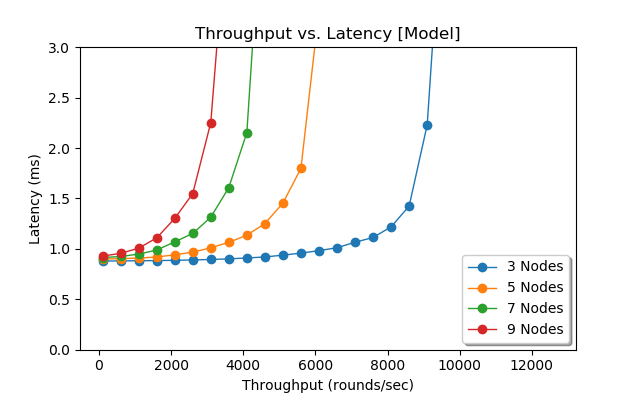Figure 4. Latency as a function of throughput for different cluster sizes (Simulation)

Next figure (Figure 5) shows how latency changes for inter-round delay variances. The runs with higher standard deviation $$\sigma_r$$ appear more “curvy”, while the runs with more uniform delay do not seem to degrade as quick until almost reaching the saturation point. High $$\sigma_r$$ runs represent more random, uncoordinated interaction with the cluster, which on my opinion is a better representation of what happens in the real world.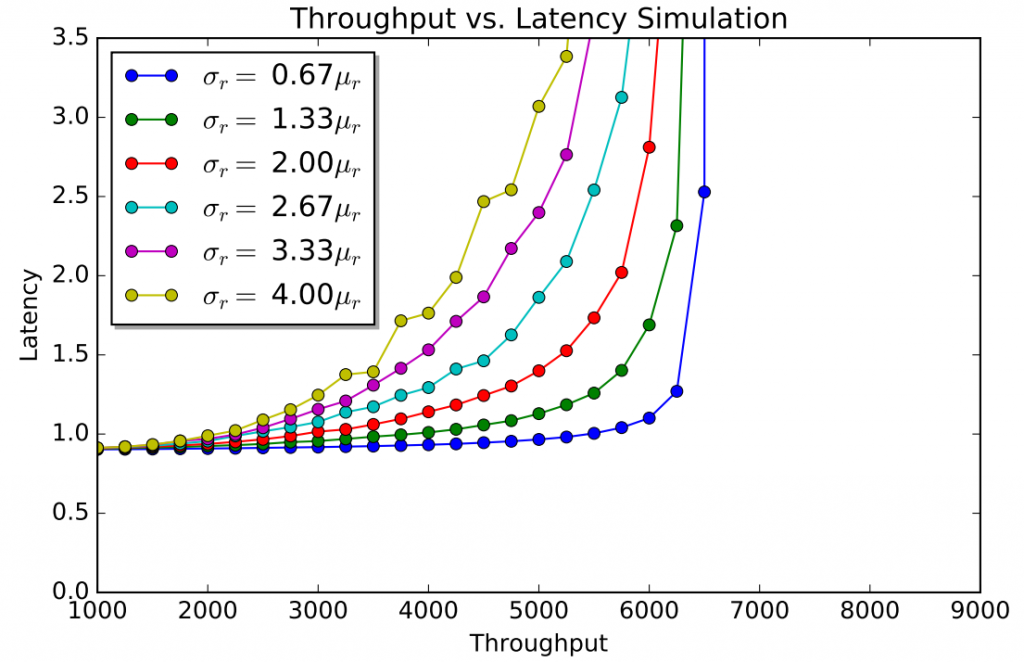Figure 5. Latency as a function of throughput in 5 node cluster for different inter-round delay parameters.

## Do I Need to Simulate Paxos Rounds?

The results above simulate many individual rounds by filling the pipeline with messages and computing the queue wait time for each round. Averaging the latencies across all simulated rounds produces the average latency for some given throughput. However, if I can compute the average queue waiting time and the average latency for the quorum message, then I no longer need to simulate individual rounds to essentially obtain these parameters. This will allow me to find the average round latency much quicker without having to repeat round formula computations over and over again.

Let’s start with computing average latency for a quorum message $$r_{lq-1}$$. Since that $$l_{q-1}$$ represents the last message needed to make up the quorum, I can model this message’s latency with some $$k$$th-order statistics sampled from Normal distribution $$\mathcal{N}(\mu_l+\mu_{ms}+\mu_{md}, \sigma_l^2 + \sigma_{ms}^2 + \sigma_{md}^2)$$ on a sample of size $$N-1$$, where $$k=q-1$$. To make things simple, I use Monte Carlo method to approximate this number $$r_{lq-1}$$ fairly quickly and accurately.

Now to approximating the queue wait time $$w_{q-1}$$. This is a bit more involved, but luckily queuing theory provides some easy ways to compute/estimate various parameters for simple queues. I used Marchal’s average waiting time approximation for single queue with generally distributed inter-arrival and service intervals (G/G/1). This approximation allows me to incorporate the inter-round interval and variance from my simulation into the queuing theory model computation.

I will spare the explanation on arriving with the formula for the average round queue wait time (it is pretty straightforward adaptation from here, with service and arrival rates expressed as rounds per second) and just give you the result for a single queue and single worker:

• $$p = R(N\mu_{md} + 2\mu_{ms})$$, where $$p$$ is queue utilization or probability queue is not busy.
• $$C_s^2 = \frac{N^2\sigma_{md}^2 + 2^2\sigma_{ms}^2}{(N\mu_{md} + 2\mu_{ms})^2}$$
• $$C_a^2 = \frac{sigma_r^2}{\mu_r^2}$$
• $$w=\frac{p^2(1+C_s^2)(C_a^2+C_s^2p^2)}{2R(1-p)(1+C_s^2p^2)}$$

With the ability to compute average queue waiting time and average time for message $$l_{q-1}$$ turn around, I can compute the average round latency time for a given throughput quickly without having to simulate multiple rounds to get the average for these parameters. $$L = 2\mu_{ms} + 2\mu_{md} + r_{lq-1} + w + \mu_l$$, where $$r_{lq-1}$$ is the mean RTT for quorum message $$l_{q-1}$$ and $$w$$ is the average queue wait time for given throughput parameters and $$\mu_l$$ is the network RTT for a message exchange with the client.

As such, the average round latency becomes:

$$L = 2\mu_{ms} + 2\mu_{md} + r_{lq-1} + \frac{p^2(1+C_s^2)(C_a^2+C_s^2p^2)}{2R(1-p)(1+C_s^2p^2)} + \mu_l$$

Figure 6 shows the model’s results for latency at various throughputs. The queuing theory model exhibits very similar patterns as the simulation, albeit the simulation seems to degrade quicker at higher throughputs then the model, especially for 3-node cluster. This may due to the fact that the simulation captures the message distribution within each round, while the model looks at the round as one whole.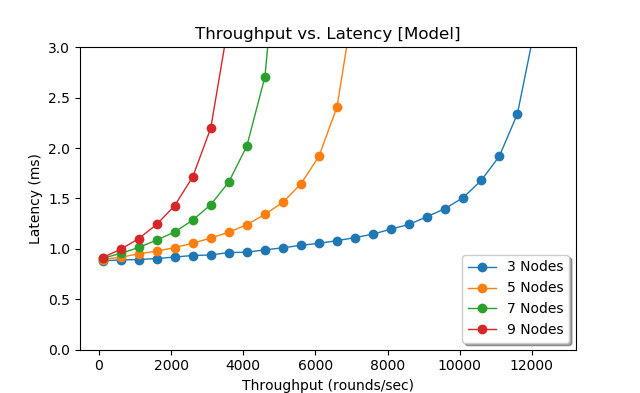Figure 6. Model for latency as a function of throughput for different cluster sizes.

## Flexible Quorums

I can use both the simulation and the model to show the difference between paxos and flexible paxos (FPaxos) by adjusting the quorums. For instance, I modeled a 9-node deployment of flexible paxos with phase-2 quorum $$q2$$ of 3 nodes. In my setup, flexible paxos must still communicate with all 9 nodes, but it needs to wait for only 2 replies, thus it can finish the phase quicker then the majority quorum. However, as seen in Figure 7, the advantage of smaller quorum is tiny compared to normal majority quorum of 9-node paxos. Despite FPaxos requiring the same number of messages as 5-node paxos setup, the costs of communicating with all 9 nodes do not allow it to get closer in performance event to a 7-machine paxos cluster.Figure 7. Modeling Paxos and FPaxos 9 nodes, with |q2|=3

## Conclusion and Next steps

So far I have modeled single-leader paxos variants in the local area network. I showed that network variations have a negligible impact on majority quorum paxos. I also illustrated that it is hard to rip the performance benefits from flexible quorums, since queuing costs of communicating with large cluster become overwhelming. However, not everything is lost for FPaxos, as it  can reduce the number of nodes involved in phase-2 communication from full cluster size to as little as $$|q2|$$ nodes and greatly mitigate the effects of queue waiting time for large clusters.

The simulation and model are available on GitHub, so you can check it out and tinker with parameters to see how the performance may change in response.

There are still quite a few other aspects of paxos that I find interesting and want to model in the future. In particular, I want to look at WAN deployments, multi-leader paxos variants and, of course, our WPaxos protocol that combines multi-leader, WAN and flexible quorums.

# Paxos Performance Modeling – Part 1.5

This post is a quick update/conclusion to the part 1. So, does the network variations make any impact at all? In the earlier simulation I showed some small performance degradation going from 3 to 5 nodes.

The reality is that for paxos, network behavior makes very little difference on scalability, and in some cases no difference at all. To see what I mean, look at the figure below: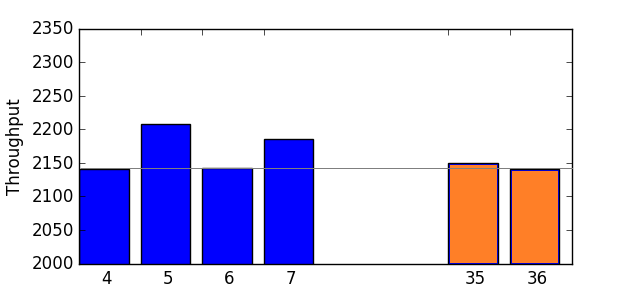See how 4 and 6 and 36 node perform the same in my simulation? And how 5, 7 and even 35 nodes clusters slightly outperforms 4 nodes?

The intuitive high level explanation for even-numbered simulation results is quite simple. For even numbered cluster sizes, a round leader receives an odd number of replies, assuming a self-voting leader. The leader also decides the round after reaching a majority  quorum $$q=\left \lfloor{\frac{N}{2}}\right \rfloor +1$$, meaning that it needs to receive $$q-1$$  or $$\left \lfloor{\frac{N}{2}}\right \rfloor$$messages (with self-voting). As it happens, for even clusters, this message is exactly the median fastest message of the round. For instance, a 6 node cluster leader will receives 5 replies, but the round reaches the majority at the 3rd (or median) reply.

Since the simulation draws message RTTs from a normal distribution, the median (50th  percentile) RTT is also the mean. After repeating it for sufficient number of rounds, any fluctuations are averaged out, resulting in an average round decided by a message with an average RTT for the network.

The cluster with odd number of servers, however, decides on the round at a message with RTT slightly less then the median RTT. This is because we have an even number of replies, and median is computed be averaging two middle RTTs. The smaller of the two values used for computing the median is actually the quorum message for the round.  For instance, in a 7-node deployment, the leader reaches quorum after receiving 3rd message $$l_3$$, with median being $$\frac{l_3+l_4}{2}$$

As a result, after many round repeats (I do ~125000 rounds) the simulation still ends up with an average RTT of a quorum message to be a tiny bit less than the median/mean RTT, and the more nodes I add, the closer it becomes to the actual 50th percentile and the mean.

So, what do we have after all of this? I think it is safe to assume the effects of network variance on paxos performance are very small and sometimes non-existent. We should not worry about the network as much, as long as it is stable and delivers predictable performance.  However, if you have a system with non-majority quorums, you may get slight benefit from quicker replies.

Update (3/10/2018):

• Part 2 – Queuing/Processing overheads

# Do not Blame (only) Network for Your Paxos Scalability Issues. (PPM Part 1)

In the past few months our lab has been doing a lot of work with different flavors of paxos consensus algorithm. Paxos and its numerous flavors are widely used in today’s cloud infrastructure. Distributed systems rely on it for many different tasks to ensure safe operation. For instance, coordination services use some consensus protocol flavor to provide services like leader election, cluster membership, service discovery and metadata management. Databases, such as Spanner or CockroachDB, use paxos to provide fault tolerant-replication of data across nodes or even datacenters.

If you work with Paxos, or had to deal with it at some point, you probably heard that it does not scale well to large number of nodes. ”Five nodes is ideal, do not try more” rhetoric has been repeated many times by many people and it gets engraved into one’s mind. ZooKeeper’s Administrator’s guide mentions 3 and 5 server deployments. Fairly recent epaxos provides evaluation on 3 and 5 node deployments in their paper. Paxos Made Live paper also mentions five nodes as typical Chubby deployment.

But why five servers is such a magical number in the Paxos world? The most common answer is along the lines of ”Increasing the number of servers increases the quorum size, making the paxos round leader wait for more messages to come to reach consensus”. This explanation is straightforward on the surface, after all, waiting for n nodes to reply should take longer than waiting for n − 1. The question then becomes why waiting for more replies is expensive? I thought the answer would be largely network related, but it appears to be more complex.

## Modeling Paxos Performance: First Attempt

To answer this, I tried to model the non-faulty paxos execution time by looking at message exchange between nodes in the cluster. In the nutshell, running a phase of paxos requires one round-trip-time (RTT) between a node and its peers: the node broadcasts the message and waits for the quorum to reply. With network arguably being the slowest part, I can try  to express the paxos phase performance through the communication delays

First, let me define some variables to model a phase of paxos:

• $$r_l$$ – message RTT in local area network
• $$\mu_l$$ – average message RTT in local area network
• $$\sigma_l$$  – standard deviation of message RTT in local area network
• $$N$$ – number of nodes participating in a paxos phase
• $$q$$ – quorum size. For a majority quorum $$q=\left \lfloor{\frac{N}{2}}\right \rfloor +1$$
• $$m_s$$ – time to serialize a message
• $$m_d$$ – time to deserialize and process a message. This involves various message-related round tasks, such as ballot comparisons, log maintenance/updated, etc.

I assume that the network performance, at least in the local area, is normally distributed. Figure 1 shows a normalized histogram (shaded area is equal to 1) of latencies of approximately 2,000 ping requests within an AWS region.

RTT $$r_l$$ for every message is drawn from a normal distribution $$\mathcal{N}(\mu_l+m_s+m_d, \sigma_l^2)$$. This distribution simulates RTTs with additional  static, non-variable delay for serialization and deserialization. As such, the only variability so far is due to the network behavior.

In order to run a round of paxos, a node needs to send $$N − 1$$ messages. That is, a node sends a message to every other node except for itself. I assume a ”leader” is also an acceptor with a short-circuit behavior, where a it does not need to send a vote to itself over a network.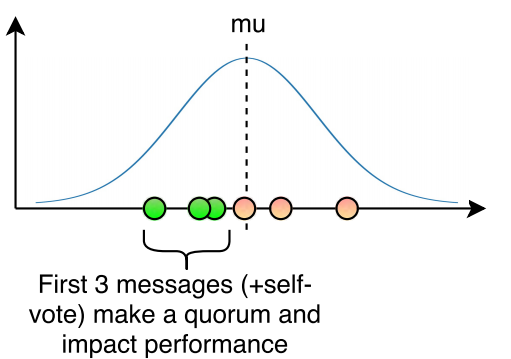Figure 2: Message RTT for a 7 node cluster

Out of the $$N − 1$$ messages sent, only $$q − 1$$ messages actually matter. Upon receiving $$q − 1$$ successful (once again, assuming non-faulty execution) replies a node has achieved a quorum and can finish the round (Figure 2). We can simulate this by drawing $$N −1$$ random RTTs $$r_{l1}, r_{l2},…, r_{lN-1}$$ from $$\mathcal{N}(\mu_l+m_s+m_d, \sigma_l^2)$$ and sorting them. The $$q − 1$$ fastest message $$r_{lq−1}$$ is the one carrying the last vote to make up a quorum, thus after processing this message, the node no longer needs to wait for other messages to come.

Assuming that a node broadcasts all messages at the same time, message RTT $$r_{lq−1}$$ can then be used to express the latency $$L_r$$ for the entire round: $$L_r = m_s + r_{lq−1} + m_d$$. Figure 3 visually represents the paxos round expressed in the formula.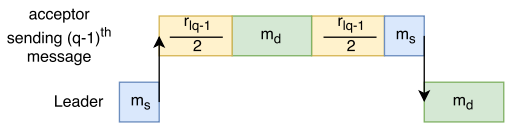Figure 3: Visual representation of time needed to execute a round

## Does the Model Make Sense?

With this simple model, I can simulate paxos execution. In multi-paxos optimization where phase-1 is used to primarily pick a stable leader and phase-2 repeats a number of times, the performance of paxos is approximated by just looking at phase-2. If $$m_s$$ and $$m_d$$ are constant, the variability in performance is only due to the network fluctuations.

I created a small python script to run such simulation, as if a single client was interacting with the paxos synchronously one command at a time. Figure 4 illustrates the results with $$m_s = m_d = 0.01 ms$$ and network parameters taken from AWS ping latency figure.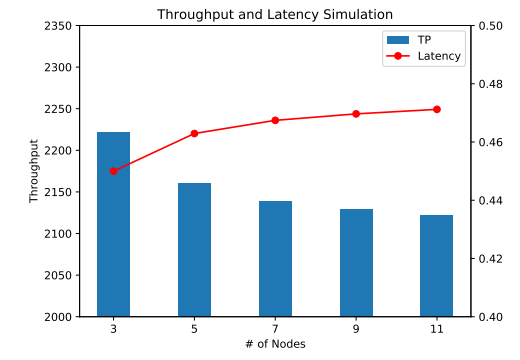Figure 4: Simulated Thtoughput and Latency from one client

At the first glance, we observe degradation in throughput and increase in latency. However, the performance decreases very slowly, and once I reach 9 nodes, the performance stays almost flat. This is very different from the data we have observed on our actual paxos implementation. Figure 5 shows the throughput and latency from a few concurrent clients interacting with the protocol.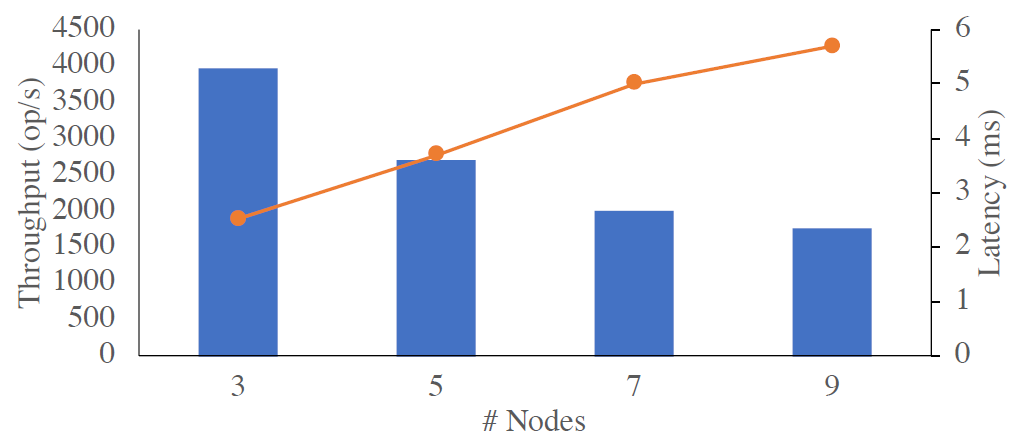Figure 5: Paxos Throughput and Latency from a small number of concurrent clients

The first thing that catches my attention is the performance degradation between the 3 and 5 node deployments. On the simulation, the difference in throughput was only 2.8%, while real paxos degraded by astonishing 30%.  As the cluster size increases, beyond 5 nodes, the real paxos also appear to degrade quicker.

Clearly, the network variability alone cannot explain the performance hit from increasing the number of nodes. So what is missing from the simple model that greatly impacts the performance as the cluster grows?

## Towards an Improved Model

Starting from the obvious, the per-message serialization $$m_s$$ and processing $$m_d$$ overheads are not static constant parameters, instead they introduce some variance as well. However, $$m_s$$ and $$m_d$$ are small to start with, and making them introduce additional variance to the model will make it better, but it will not change the overall model simulation much.

Assuming that $$m_s$$ is drawn from a normal distribution $$\mathcal{N}(\mu_{ms}, \sigma_{ms}^2)$$ and $$m_d$$ is similarly drawn from $$\mathcal{N}(\mu_{md}, \sigma_{md}^2)$$, then the message RTT time $$r_{l}$$ must be drawn from $$\mathcal{N}(\mu_l+\mu_{ms}+\mu_{md}, \sigma_l^2 + \sigma_{ms}^2 + \sigma_{md}^2)$$. Making this changes to the model resulted in a difference between 3 and 5 node simulations to grow from 2.8% to 2.9% with $$\mu_{ms} = \mu_{md} = 0.01$$ and $$\sigma_{ms} = \sigma_{md} = 0.002$$ (some arbitrary values that make little impact, unless same magnitude as network mean and standard deviation).

Introducing the variability to message overheads $$m_s$$ and $$m_d$$ still does not account for these overheads being dependent on the number of node in the cluster. The dependence on $$N$$ is not direct, and instead the per-message costs increase as the number of messages that a server needs to handle grows. This puts a ”leader” node repeating the phase-2 of paxos in a more vulnerable position as it will experience the increased traffic.

To understand how message serialization and processing depends on the number of messages exchanged, we need to consider the implementation of application’s network layers. Often times, as an application receives the message, it will use one or more processing queues or pipelines to deserialize the message and dispatch it to the appropriate handler for processing.

Let’s consider an example with just one such pipeline. If the queue is empty upon message arrival, it will get to deserialize with no further delay. However, when there are other messages in the queue, the new message has to wait for it turn to be processed.

A single Paxos round has a bursty network utilization, especially at the leader. This is because the leader node first broadcasts a messages and then receives multiple replies at roughly the same time. If the message deserialization has not finished before the next message arrives, then the pipeline gets clogged. Further messages potentially make the issue worse by growing the queue, as shown in Figure \ref{fig:pipeline_clogged}.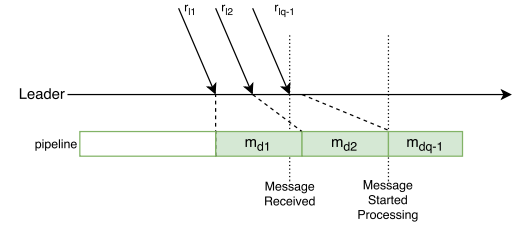Figure 6: Example of deserialization pipeline stalling paxos round

Obviously, having multiple parallel pipelines can help, given that they are balanced and there are enough compute resources to run them. However, the problem can also get worse when we start running rounds concurrently (i.e. multiple clients interact with paxos). In such scenario, messages from different rounds will compete for the fixed number of processing queues.

Where does this leave me with trying to model paxos round performance? I need to account for a number of additional factors, such as the number of message-processing queues/pipelines/threads, the rate of message deserialization at each queue, and the number of concurrent paxos rounds. Oh, and since the messages arriving after the quorum has been reached still need to get deserialized, they will contribute to how clogged the pipelines are.

I will pick up from this point onwards in some future post. I will also try to look at flexible quorums and our WPaxos protocol.

Update (3/10/2018):

• Part 1.5 – Does network matter at all?
• Part 2 – Queuing/Processing overheads

# EPaxos: Consensus with no leader

In my previous post I talked about Raft consensus algorithm. Raft has a strong leader which may present some problems under certain circumstances, for example in case of leader failure or when deployed over a wide area network (WAN). Egalitarian Paxos, or EPaxos, discards the notion of a leader and allows each node to be a leader for the requests it receives. “There Is More Consensus in Egalitarian Parliaments“ talks about the algorithm in greater details.

According to the designers of the algorithm, they were trying to achieve the following three goals:

• Good commit latency in WAN
• Good load balancing
• Graceful performance decrease due to slow or failing replicas

These goals are achieved by decentralized ordering of commands. Unlike classical Paxos and many Paxos-lie solutions, EPaxos has no central leader, and orders the commands dynamically and only when such ordering is required.  Upon receiving a request, EPaxos replica becomes a request-leader, it then uses fast quorum to establish any dependencies for such request. If no dependencies are found, it can proceed to committing a request, but if the request conflicts with other requests being processed, the order of the request is established taking into account all the dependencies in a manner similar to regular Paxos algorithm.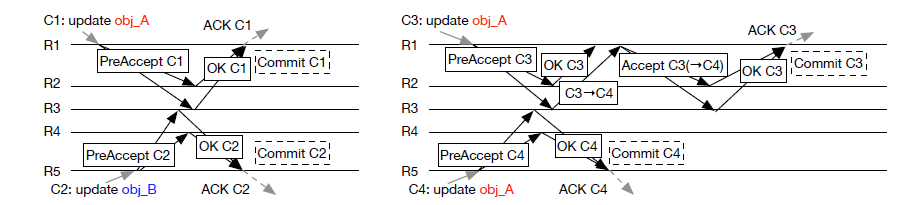Figure 1. Sample Message flow in EPaxos. R1-R5 are replicas, obj_A and obj_B are requests. Arrows designate message flow. Figure taken from the “There Is More Consensus in Egalitarian Parliaments” paper.

The figure above illustrates two scenarios. On the left we have two requests coming in to different EPaxos nodes (R1 and R5), and such requests have no dependencies on each other, so both R1 and R5 can proceed to commit right after the fast quorum tells them there are no dependencies. Similarly, on the right side we have two messages arriving from the client to their corresponding replicas, but Message C3 has a dependency on message C4. R1 learns of such dependency through node R3 and must invoke Paxos accept stage of the algorithm to enforce proper ordering of the requests, as such we order C3 only after C4 and commit C3 after C4 has been committed and executed.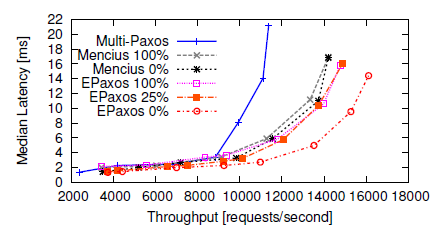Figure 2. Latency vs Throughput. Figure taken from the “There Is More Consensus in Egalitarian Parliaments” paper.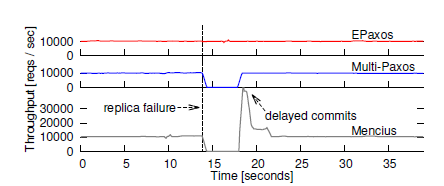Figure 3. Throughput in case of a replica failure (leader failure for Multi-Paxos). Figure taken from the “There Is More Consensus in Egalitarian Parliaments” paper.

EPaxos demonstrates good performance and stability when compared to other common consensus algorithms. Both figures above are from the EPaxos paper mentioned earlier. It is interesting to see how throughput stays the same for EPaxos in the event of a node failure, while classical Multi-Paxos virtually stops until a new leader can be elected. In Figure 2, the percentage next to the algorithm name designates the conflicting commands, or commands that have dependencies. As can be seen EPaxos generally performs well, even with a high number of conflicting commands, although the difference between 25% and 100% conflicting commands seems small. It is worth noting that based on previous and related work, authors estimate 2% conflicting commands in real world situations.